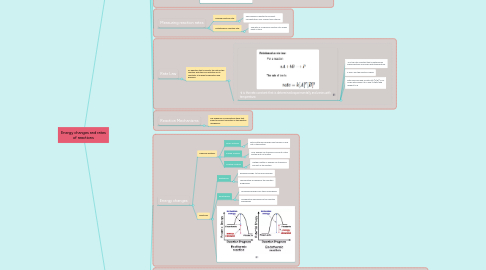# Energy changes and rates of reactions

Organic Chemistry Concept Map by Haya Tariq.

Get Started. It's FreeEnergy changes and rates of reactions## 1. Thermochemistry

### 1.1. Energy changes

1.1.1. Chemical systems

1.1.1.1. Open Systems

1.1.1.1.1. Both matter and energy may transfer in and out of the system.

1.1.1.2. Closed Systems

1.1.1.2.1. Only energy can transfer in and out of the system but not matter.

1.1.1.3. Isolated Systems

1.1.1.3.1. Neither matter or energy can transfer in and out of the system.

1.1.2. Reactions

1.1.2.1. Exothermic

1.1.2.1.1. Releases energy to the surroundings.

1.1.2.1.2. Temperature increases as the reaction progresses.

1.1.2.2. Endothermic

1.1.2.2.1. Absorbing energy from the surroundings.

1.1.2.2.2. Temperature decreases as the reaction progresses

1.1.2.3. i

### 1.2. Calorimetry and Enthalpy

1.2.1. Calculations

1.2.1.1. Finding Thermal energy

1.2.1.1.1. i

1.2.1.2. Finding Enthalpy change

1.2.1.2.1. Enthalpy change of a chemical system is equal to the flow of thermal energy in and out of the system.

1.2.1.3. Finding molar enthalpy change

1.2.1.3.1. ΔH =nΔHr

### 1.3. Bond energies

1.3.1. Calculations

1.3.1.1. i

1.3.1.2. Finding Enthalpy change

1.3.1.2.1. Enthalpy change can be estimated by finding the quantity of energy associated with the chemical bonds of both the reactants and products.

### 1.4. Hess's Law

1.4.1. States that the enthalpy change for the conversion of reactants to products is the same whether the conversion occurs in one step or multiple processes.

1.4.1.1. i

### 1.5. Standard Enthalpies of Formation

1.5.1. It is the enthalpy change that occurs in 1 mole of a substance formed in its standard state at a specific temperature from stable forms of its elements.

1.5.1.1. Calculation:

1.5.1.1.1. To find standard enthalpy of formation, Use a standard enthalpies of formation table, and using those values , standard enthalpies of formation can be found.

### 1.6. Present and future energy sources

1.6.1. Conventional fuels

1.6.1.1. Fossil fuels

1.6.1.1.1. Non-renewable energy sources

1.6.1.2. Nuclear energy

1.6.1.2.1. Nuclear energy is the energy held in the nucleus of an atom.

1.6.2. Alternative energy sources

1.6.2.1. Synthethic coal-based fuels

1.6.2.2. Hydrogen gas fuel

1.6.2.2.1. Hydrogen fuel is a zero-emission fuel burned with oxygen.

1.6.2.3. Biofuels

1.6.2.3.1. Any fuel that is derived from biomass

1.6.3. Energy efficiency

## 2. Chemical Kinetics

### 2.1. Explaining reaction rates

2.1.1. Collison theory

2.1.1.1. States that chemical reactions can occur only when reacting particles collide with each other in a specific way and with sufficient energy.

2.1.1.1.1. f

2.1.1.1.2. Activation energy:

2.1.1.1.3. Orientation

### 2.2. Factors affecting reaction rates:

2.2.1. Chemical nature of reactants

2.2.1.1. The rate of any reaction depends on the chemical nature and the physical properties of the substances.

2.2.2. Concentration of reactants

2.2.2.1. An increase in reactant concentration increases the rate of reaction.

2.2.2.1.1. Increasing concentration increases the number of collisions hence increasing the number of effective collisions.

2.2.3. Surface area

2.2.3.1. Increasing the surface area of a solid reactant increases the reaction rate. as only atoms and ions at the surface of the reactant can interact with other reactants.

2.2.3.1.1. Increasing surface area increases the number of collisions hence increasing the number of effective collisions.

2.2.4. Temperature of the reaction system

2.2.4.1. An increase in temperature increases the rate of a reaction as it gives the particles more energy to collide.

2.2.4.1.1. Increasing temperature will increase the average kinetic energy of the reactants, increasing number of effective collisions.

2.2.5. Presence of a catalyst

2.2.5.1. Catalysts increase the reaction rates of a reaction without having itself consumed in the reaction.

2.2.5.1.1. This provides a reaction pathway to an effective collision with a lower activation energy.

2.2.6. n

### 2.3. Measuring reaction rates

2.3.1. Average reaction rate:

2.3.1.1. The change in reactant or product concentration over a given time interval.

2.3.2. Instantaneous reaction rate:

2.3.2.1. The rate of a chemical reaction at a single point in time.

### 2.4. Rate Law

2.4.1. An equation that connects the rate of the reaction with the concentration of its reactants at a given temperature and pressure.

2.4.1.1. -k is the rate constant that is determined experimentally and varies with temperature.

2.4.1.1.1. -k is the rate constant that is determined experimentally and varies with temperature.

2.4.1.1.2. x and y are the reaction orders

2.4.1.1.3. Rate laws are read as rate=k[A]^x[B]^y is x order with respect to A and y order with respect to B

### 2.5. Reaction Mechanisms

2.5.1. The sequence of elementary steps that leads to product formation is the reaction mechanism.